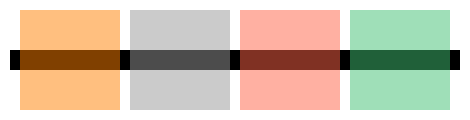# Alpha colour in generativepy

Martin McBride, 2021-11-10
Tags generativepy tutorial colour alpha
Categories generativepy generativepy tutorialYou can transparent colours by adding an extra parameter, a, which has a value between 0.0 (totally transparent, ie invisible) and 1.0 (fully opaque). This can be done with any of the above methods:

• RGB colours: Color(r, g, b, a)
• Grey colours: Color(k, a)
• CSS colours: Color(name, a)
• HSL colours: Color.of_hsla(h, s, l, a) (note that this is of_hsla rather than of_hsl).

The code below creates a set of 50% transparent coloured squares, using the four functions above. The code first draws a black bar across the image and paints the transparent colours over the top, so you can see the bar through the squares.

In this example, we have left the background white so that the background colour doesn't show through the transparent squares.

from generativepy.drawing import make_image, setup
from generativepy.color import Color
from generativepy.geometry import Rectangle

def draw_alpha(ctx, pixel_width, pixel_height, frame_no, frame_count):
setup(ctx, pixel_width, pixel_height, background=Color(1))

Rectangle(ctx).of_corner_size((10, 50), 450, 20).fill(Color(0))

pos = [20, 10]
w = 100
space =110
h = 100

Rectangle(ctx).of_corner_size(pos, w, h).fill(Color(1.0, 0.5, 0.0, 0.5))
pos += space
Rectangle(ctx).of_corner_size(pos, w, h).fill(Color(0.6, 0.5))
pos += space
Rectangle(ctx).of_corner_size(pos, w, h).fill(Color("tomato", 0.5))
pos += space
Rectangle(ctx).of_corner_size(pos, w, h).fill(Color.of_hsla(0.4, 0.5, 0.5, 0.5))
pos += space

make_image("colour-alpha.png", draw_alpha, 470, 120)


This code is available on github in tutorial/colour/colour_alpha.py.

Here is the image it creates:If you found this article useful, you might be interested in the book Computer Graphics in Python or other books by the same author.

#### Popular tags

2d arrays abstract data type alignment and angle animation arange arc array arrays behavioural pattern bezier curve built-in function callable object chain circle classes clipping close closure cmyk colour combinations comparison operator comprehension context context manager conversion count creational pattern data science data types design pattern device space dictionary drawing duck typing efficiency ellipse else encryption enumerate fill filter font font style for loop function function composition function plot functools game development generativepy tutorial generator geometry gif global variable gradient greyscale higher order function hsl html image image processing imagesurface immutable object in operator index inner function input installing iter iterable iterator itertools join l system lambda function len line linear gradient linspace list list comprehension logical operator lru_cache magic method mandelbrot mandelbrot set map matplotlib monad mutability named parameter numeric python numpy object open operator optimisation optional parameter or pandas partial application path pattern permutations polygon positional parameter print pure function python standard library radial gradient range recipes rectangle recursion reduce repeat rgb rotation roundrect scaling scipy sector segment sequence setup shape singleton slice slicing sound spirograph sprite square str stream string stroke structural pattern subpath symmetric encryption template text text metrics tinkerbell fractal transform translation transparency triangle truthy value tuple turtle unpacking user space vectorisation webserver website while loop zip